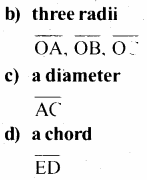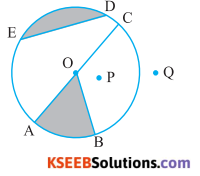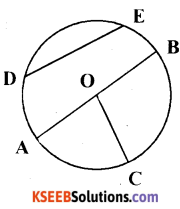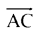# KSEEB Solutions for Class 6 Maths Chapter 4 Basic Geometrical Ideas Ex 4.6

Students can Download Chapter 4 Basic Geometrical Ideas Ex 4.6 Questions and Answers, Notes Pdf, KSEEB Solutions for Class 6 Maths helps you to revise the complete Karnataka State Board Syllabus and score more marks in your examinations.

## Karnataka State Syllabus Class 6 Maths Chapter 4 Basic Geometrical Ideas Ex 4.6

Question 1.
From the figure, identify:
Solution:
a) the centre of circle
Oe) a sector
O.P

f) a segment
Q

g) a sector

h) a segmentQuestion 2.
a) Is every diameter of a circle also a chord?
Solution:
Yes, the diameter is the longest possible chord of the circle

b) Is every chord of a circle also a diameter?
Solution:
No.

Question 3.
Draw any circle and mark
Solution:
a) its centre
O$$\overrightarrow{\mathrm{OA}}$$

c) a diameter
$$\overrightarrow{\mathrm{AB}}$$

d) a sector
COAe) a segment
DE

f) a point in its interior
O

g) a point in its exterior
F

h) an arcQuestion 4.
Say true or false
Solution:
a) Two diameters of a circle win necessarily intersect
True, They will along interest each other at the centre of the circle

b) The centre of a circle is always in its interior.
True